Next: A.4 Capacitance of an Up: A Capacitance Calculations Previous: A.2 Self Capacitance of

# A.3 Capacitance of Two Spheres

First we study the case of a point charge near a grounded conducting sphere by the  image charge method. Removing the conductor we try to find the position and magnitude of an image' charge q', as in Fig. A.2, that will make the potential zero on the spherical surface.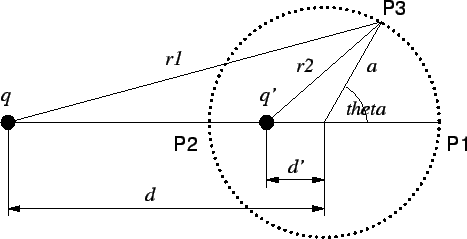It is clear from the symmetry of the problem, that if such a charge exists it must lie on the line connecting q and the center of the sphere. We begin by forcing the potential to vanish at the points P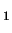and P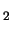. Then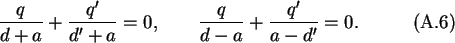Solving these two equations gives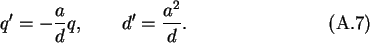We still have to assure whether this charge arrangement will make the potential zero at a general point P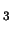on the surface of the sphere. At P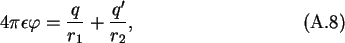where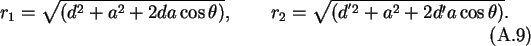Taking q' and d' as in (A.7) does in fact make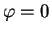at P, as required. Since this set of charges has a rotation symmetry around the axis P-P, the condition is indeed fulfilled for all points on the sphere.

Applying the above result for a successive approximation in a method of images, one can calculate the capacitance of two spheres, which is applied here for two equal spheres. We are replacing both spheres by a set of point charges which will maintain the conductor surfaces as equipotentials.

First we put a charge q at the center of the left sphere, as in Fig. A.3.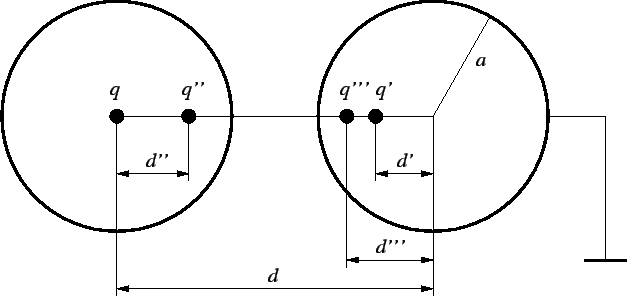This makes the left sphere an equipotential, but not the right sphere. Next we put the image' charge q' in the right sphere. This makes the right sphere a zero equipotential but destroys the spherical potential on the left. So we put the image' of the image' q'' inside the left sphere, to compensate for q'. This makes the left sphere again an equipotential but upsets the right sphere. We continue the process which converges rapidly, until we reach the required precision. The total charge on the left sphere is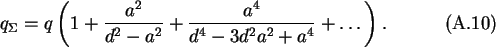But only q contributes to its potential. The charges q' and q'' cause the potential, they contribute to the left sphere, to vanish, and the same is true of all following pairs of charges. The potential of the left sphere is therefore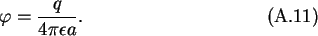The charges q and q' have a zero contribution to the potential of the right sphere and so do the pairs q'', q''' a.s.o. Since the potential of the right sphere was always compensated to zero the total capacitance of either sphere is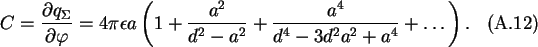The total capacitance C can be split in a capacitance between the spheres C12 and a capacitance to infinity or self-capacitance of the sphere C10, as shown in Fig. A.4.

Table A.1: Charges and location of successive compensation charges.
 left sphere right sphere charge distance charge distance from center from center q 0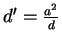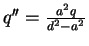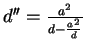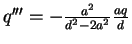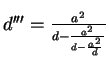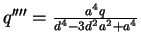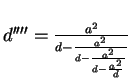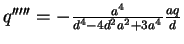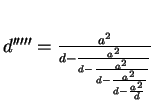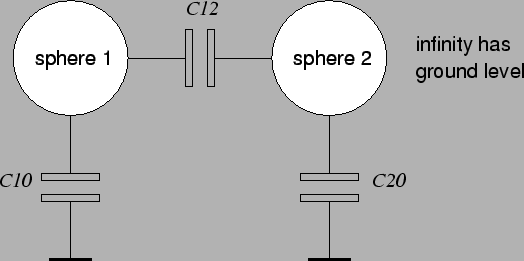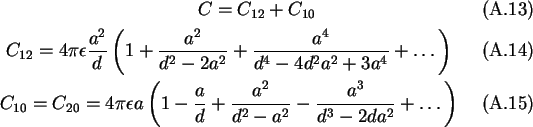Next: A.4 Capacitance of an Up: A Capacitance Calculations Previous: A.2 Self Capacitance of

Christoph Wasshuber